## Equivalent Circuit of Long Transmission Line | π and T network:

So far as the end conditions are concerned, the Equivalent Circuit of Long Transmission Line can be established in the form of a T- or π-network.

The parameters of the equivalent network are easily obtained by comparing the performance equations of a π-network and a transmission line in terms of end quantities.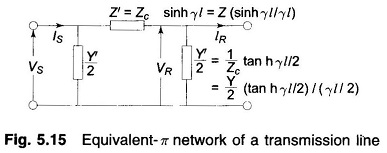For a π-network shown in Fig. 5.15.According to exact solution of a long lineFor exact equivalence, we must have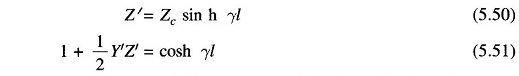From Eq. (5.50)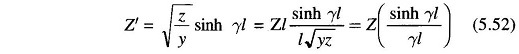Thus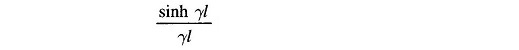is the factor by which the series impedance of the nominal-π must be multiplied to obtain the Zparameter of the equivalent-π, Substituting Z’ from Eq. (5.50) in Eq. (5.51), we get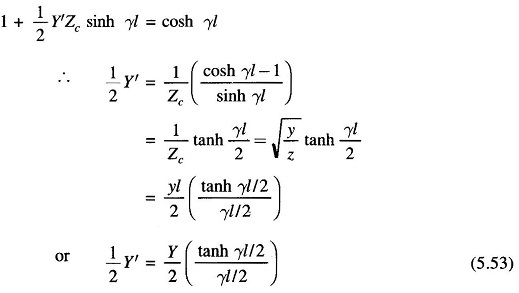Thus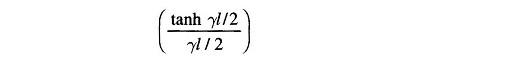is the factor by which the shunt admittance arm of the nominal- π must be multiplied to obtain the shunt parameter ( Y’/2) of the equivalent- π.

Note that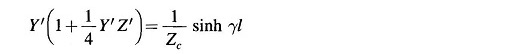is a consistent equation in terms of the above values of Y’ and Z’.

For a line of medium length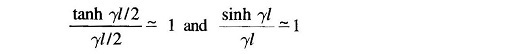so that the equivalent- π network reduces to that of nominal- π.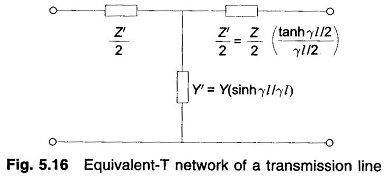Equivalent-T network parameters of a transmission line are obtained on similar lines. The equivalent-T network is shown in Fig. 5.16.

Scroll to Top